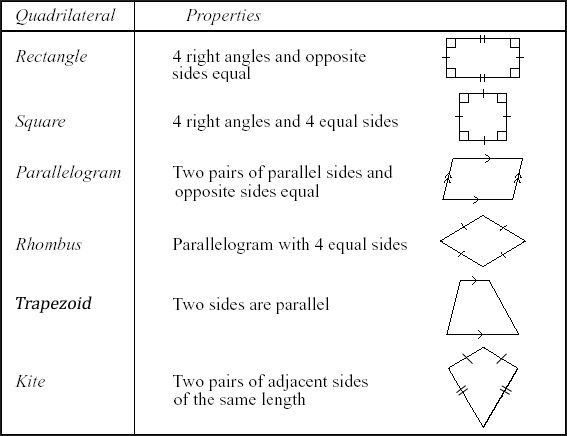Notice: Undefined index: options in /home/u337987201/domains/classnotes.ng/public_html/wp-content/plugins/elementor-pro/modules/theme-builder/widgets/site-logo.php on line 192

Welcome to JSS2 Second Term!

In today’s class, We will be discussing QUADRILATERALS. We hope you enjoy the class!

A quadrilateral is a plane figure that has four sides or edges, so having four corners or vertices. Quadrilaterals will typically be of standard shapes with four sides like rectangle, square, trapezoid, and kite or irregular and uncharacterized as shown below:There are many types of quadrilaterals. As the word ‘Quad’ means four, all these types of a quadrilateral have four sides and the sum of angles of these shapes is 360 degrees.

1. Trapezium
2. Parallelogram
3. Squares
4. Rectangle
5. Rhombus
6. KiteAnother way to classify the types of quadrilaterals are:

1. Convex Quadrilaterals: Both the diagonals of a quadrilateral are completely contained within a figure.
2. Concave Quadrilaterals: At least one of the diagonals lies partly are entirely outside of the figure.A quadrilateral is a 4-sided plane figure. Below are some important properties of quadrilaterals:

• Every quadrilateral has 4 vertices, 4 angles, and 4 sides
• The total of its interior angles = 360 degrees
• A quadrilateral with four equal sides and four right angles is a square
• A quadrilateral that has opposite sides equal and measure of every angle is 90 degrees is a rectangle
• Parallelogram, trapezium, rhombus, and kite are other examples of quadrilateralsLet us understand in a better way with the help of an example:

• Four sides: AB, BC, CD, and DA
• Four vertices: Points A, B, C, and D
• Four angles: ∠ABC, ∠BCD, ∠CDA, and ∠DAB
• ∠A and ∠B are adjacent angles
• ∠A and ∠C are the opposite angles
• AB and CD are the opposite sides
• AB and BC are the adjacent sides

What is the area of the quadrilateral?

Area of the quadrilateral is the total space occupied by the figure. There are different types of quadrilaterals. Let us study area formula each of them:

 Area of a Parallelogram Base x Height Area of a Rectangle Length x Width Area of a Square Side x Side Area of a Rhombus 1/2 x Diagonal 1 x Diagonal 2 OR Length x Height Area of a Kite 1/2 x Diagonal 1 x Diagonal 2We have come to the end of this class. We do hope you enjoyed the class?

Should you have any further question, feel free to ask in the comment section below and trust us to respond as soon as possible.

In our next class, we will be talking about Plane figures Polygons. We are eager to meet you there.

For more class notes, videos, homework help etc download our Mobile App HERE

Join ClassNotes.ng Telegram Community HERE for exclusive content and support

Don`t copy text!